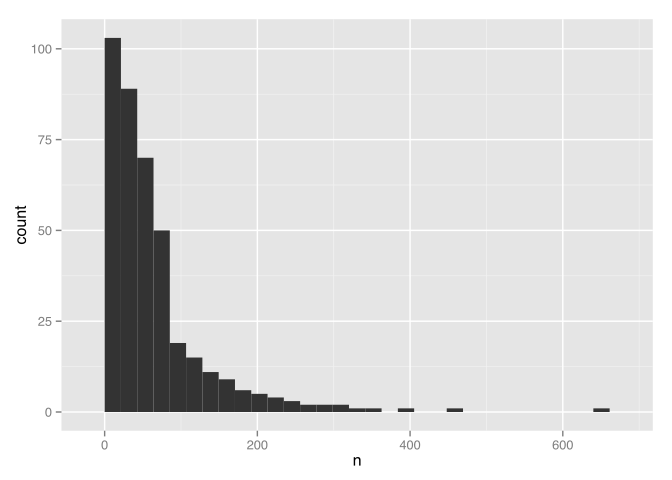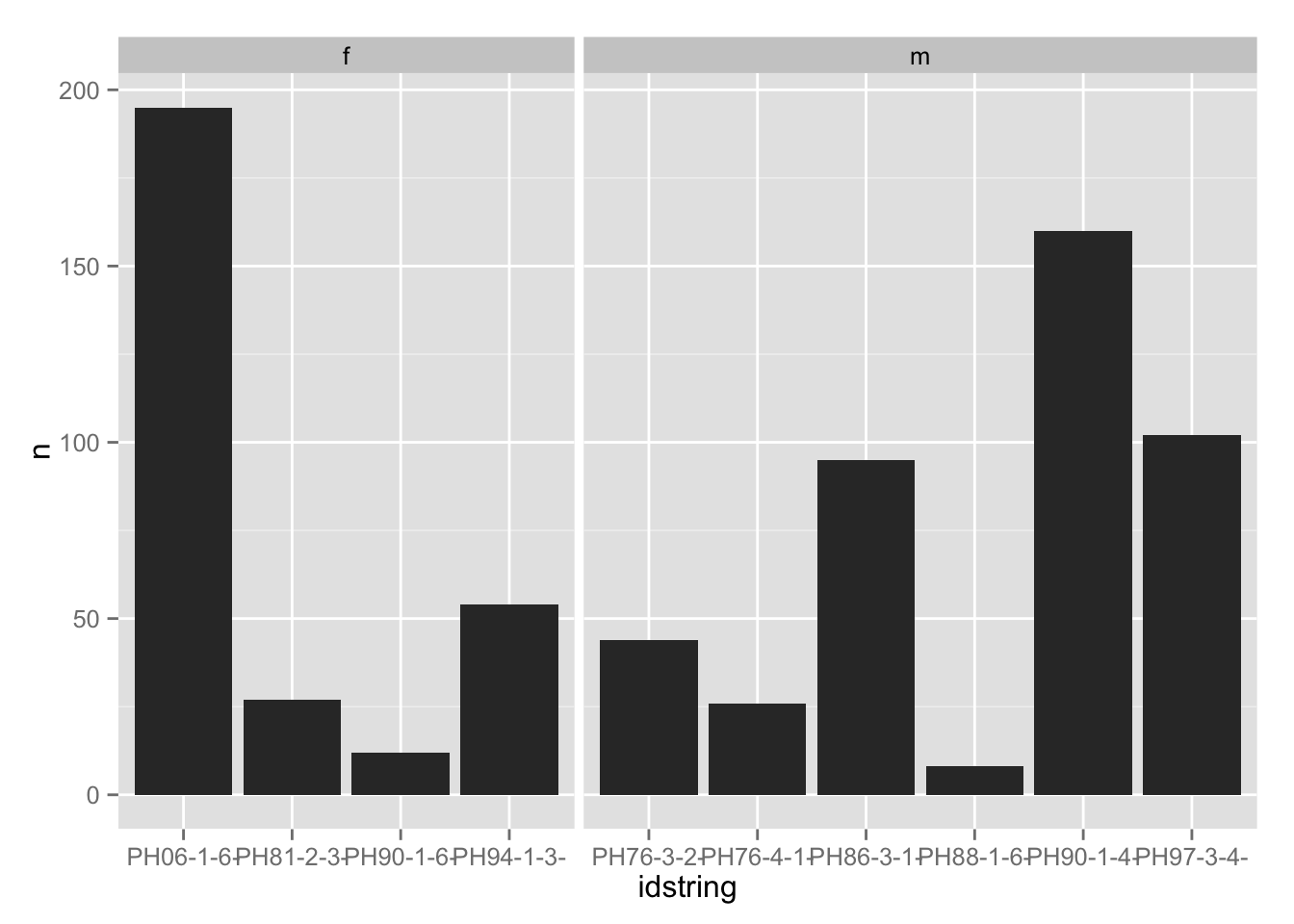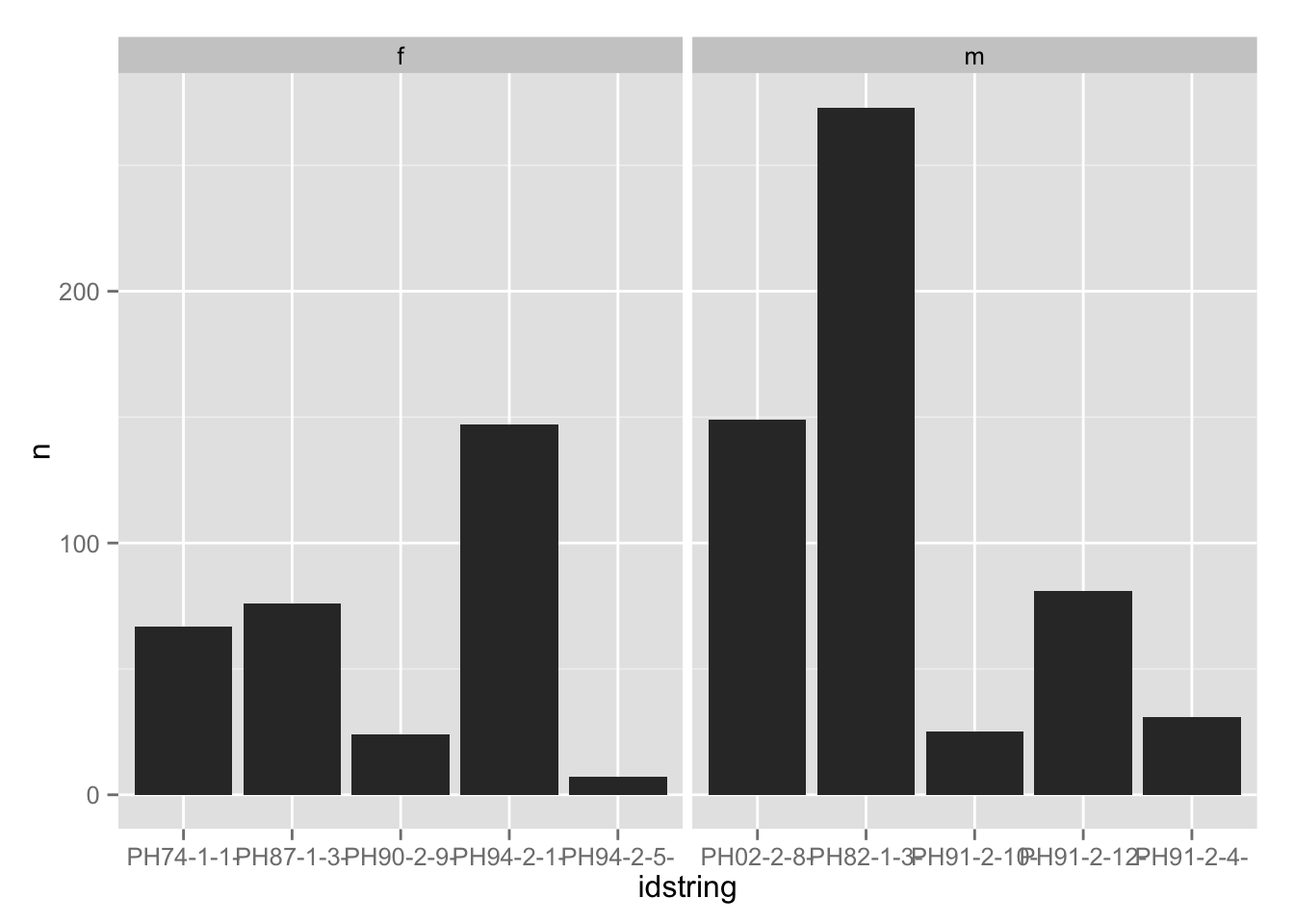This is a brief tutorial on how to subsample groups from a data frame using `dplyr`.

``````library(devtools)
install_github("jofrhwld/UhUm", quiet = T)
library(UhUm)
library(dplyr)
library(ggplot2)``````

`um_PNC` has every token of UH and UM from a collection of recorded speech called the Philadelphia Neighborhood Corpus (PNC). `idstring` corresponds to a unique id for each speaker.

``  head(um_PNC)``
``````##    idstring word start_time end_time vowel_start vowel_end nasal_start
## 1 PH00-1-1-   UH     24.388   24.688      24.388    24.688          NA
## 2 PH00-1-1-   UH     34.963   35.243      34.963    35.243          NA
## 3 PH00-1-1-   UM     37.903   38.273      37.903    38.123      38.123
## 4 PH00-1-1-   UH     44.453   44.653      44.453    44.653          NA
## 5 PH00-1-1-   UH     57.648   57.828      57.648    57.828          NA
## 6 PH00-1-1-   UH     62.298   62.487      62.298    62.487          NA
##   nasal_end next_seg next_seg_start next_seg_end chunk_start chunk_end
## 1        NA        S         24.688       24.867      24.388    25.288
## 2        NA        F         35.243       35.353      34.963    37.113
## 3    38.273       sp         38.273       38.393      37.903    38.803
## 4        NA       DH         44.653       44.682      44.453    45.623
## 5        NA      AY1         57.828       57.878      57.648    58.968
## 6        NA       sp         62.487       62.978      62.068    62.487
##   nwords sex year age ethnicity schooling transcribed    total nvowels
## 1   6551   m 2000  21       i/r        14    2810.733 2814.345    3078
## 2   6551   m 2000  21       i/r        14    2810.733 2814.345    3078
## 3   6551   m 2000  21       i/r        14    2810.733 2814.345    3078
## 4   6551   m 2000  21       i/r        14    2810.733 2814.345    3078
## 5   6551   m 2000  21       i/r        14    2810.733 2814.345    3078
## 6   6551   m 2000  21       i/r        14    2810.733 2814.345    3078``````
``````  um_PNC %>%
group_by(idstring)%>%
tally()%>%
ggplot(aes(n))+
geom_bar()``````
``## stat_bin: binwidth defaulted to range/30. Use 'binwidth = x' to adjust this.``## Subsampling

If you wanted to examine just random sample of 10 speakers from this whole data frame, the steps are:

• define the groups that will be sampled
• summarise, in any way (here, I’ll use `tally() %>% select(-n)`)
• sample from the summary using `sample_n` or `sample_frac`
• `left_join` the sample with the original data frame.
``````  subsamp_10 <- um_PNC %>%
group_by(idstring) %>%
tally() %>% select(-n) %>%
sample_n(size = 10, replace = F)%>%
left_join(um_PNC)``````
``## Joining by: "idstring"``
``````  subsamp_10 %>%
group_by(idstring, sex)%>%
tally()%>%
ggplot(aes(idstring, n))+
geom_bar(stat = 'identity')+
facet_grid(.~sex, scales = "free_x", space = "free_x")``````## Balanced across subgroups

If you want to ensure an equal number of men and women in the subsample, group by `sex` as well.

``````  subsamp_10_balanced <-  um_PNC%>%
group_by(sex, idstring)%>%
tally() %>% select(-n)%>%
sample_n(size = 5, replace = F)%>%
left_join(um_PNC)``````
``## Joining by: c("sex", "idstring")``
``````  subsamp_10_balanced %>%
group_by(idstring, sex)%>%
tally()%>%
ggplot(aes(idstring, n))+
geom_bar(stat = 'identity')+
facet_grid(.~sex, scales = "free_x", space = "free_x")``````## Proportional subgroups

If you want the sub sample to have the same gender ratio as the full sample, use `sample_frac`

``````  subsamp_prop <- um_PNC %>%
group_by(sex, idstring)%>%
tally() %>% select(-n) %>%
sample_frac(size = 0.03, replace = F) %>%
left_join(um_PNC)``````
``## Joining by: c("sex", "idstring")``
``````  subsamp_prop %>%
group_by(idstring, sex)%>%
tally()%>%
ggplot(aes(idstring, n))+
geom_bar(stat = 'identity')+
facet_grid(.~sex, scales = "free_x", space = "free_x")``````